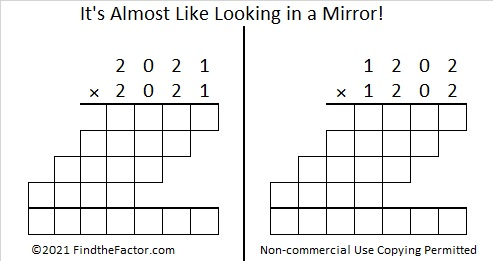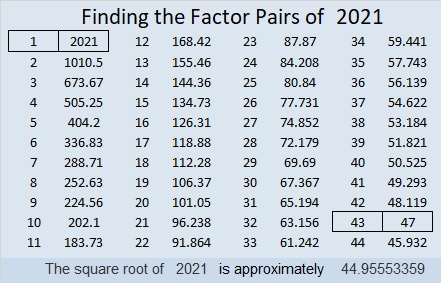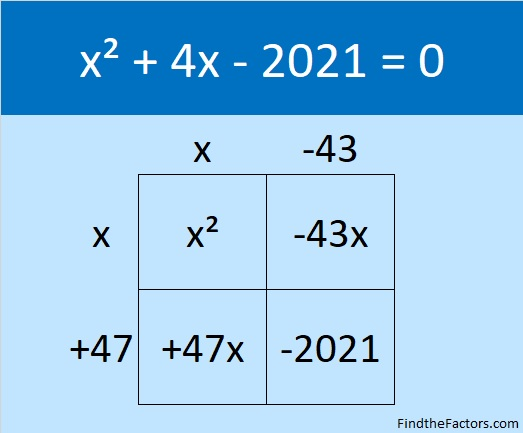# Factors and Facts for the Year 2021

Contents

### Countdown to 2021:

Here is a countdown you can use on New Year’s Eve to bring in 2021:make science GIFs like this at MakeaGif

### It’s Almost Like 2021 Is Looking in a Mirror!

Squaring means multiplying a number by itself. Something unusual happens when you square 2021 and you also square its reverse, 1202. Complete the two multiplication problems below to see for yourself!There are only 50 numbers less than 10000 with this same property. They are all listed in the table below:For each pair of numbers in the table, the smaller number was listed first, but if you look carefully you will notice 2001, 2011, 2012, 2021, and 2022 in this century and 2101, 2102, 2111, and 2121 in the next.

### Math Facts about the Number 2021:make science GIFs like this at MakeaGif

2021 is the difference of two squares in two different ways:
45² – 2² = 2021 and
1011² – 1010² = 2021.

2021 is not the hypotenuse of any Pythagorean triples, but it is a leg in four:
180-2021-2029, calculated from 2(45)(2), 45² – 2², 45² + 2²,
2021-43428-43475, which is (43-924-925) times 47,
2021-47472-47515, which is 43 times (47-1104-1105)
2021-2042220-2042221, from 1011² – 1010², 2(1011)(1010), 1011² + 1010².### Factors of 2021:

The previous year had many negative factors, although it had just as many positive ones. I can predict that 2021 will have only four negative factors to go along with its four positive ones. I think we will see a lot of the negative factors at the beginning of the year, but hopefully, the positive ones will be more evident as the year goes on.

• 2021 is a composite number.
• Prime factorization: 2021 = 43 × 47.
• 2021 has no exponents greater than 1 in its prime factorization, so √2021 cannot be simplified.
• The exponents in the prime factorization are 1 and 1. Adding one to each exponent and multiplying we get (1 + 1)(1 + 1) = 2 × 2 = 4. Therefore 2021 has exactly 4 factors.
• The factors of 2021 are outlined with their factor pair partners in the graphic below.